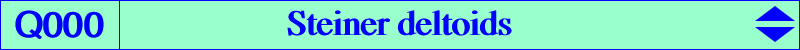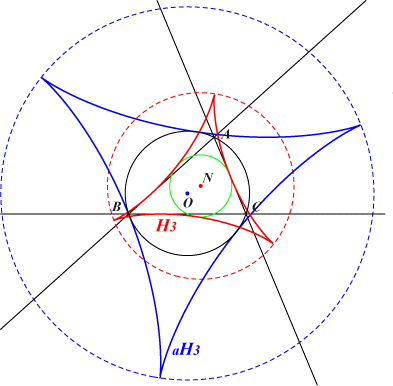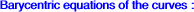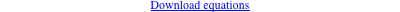too complicated to be written here. Click on the link to download a text file.H3 : ∑ a^2 (v + w) / (v - w) = 0 (1) aH3 : ∑ a^2 u / (v - w) = 0 (2) meaning that the line u x + v y + w z = 0 is tangent to the curve. Note that (1) is the barycentric equation of K010 which is the dual of H3. See also K244.on H3 : X(1553), X(6070), X(6071), X(6072), X(6073), X(6074), X(6075), X(6076), X(6077), X(14499) up to X(14507), vertices of the cevian triangle of X(69) on aH3 : X(14480), X(14508) up to X(14515), vertices of the circumtangential triangleThe Steiner deltoid H3 (envelope of Simson lines) and its anticomplement aH3 (envelope of axis of inscribed parabolas) are two very famous curves in triangle geometry. There's no question of reconsidering here their very numerous properties. We will only point out their connection with some cubics or other curves.cubic notes K071 contains the cusps of H3, a stelloid K077 contains the cusps of aH3, a stelloid K244 contains the cusps of H3 and those of any deltoid inscribed in ABC, an acnodal cubic contains the contacts of H3 with the nine point circle, an equilateral cubic K583 contains the contacts of the deltoids above with their incircle, a nodal cubic contains the cusps of H3, a circular cubic contains the cusps of H3, K650 contains the cusps of H3, contains the cusps of H3, contains the cusps of H3, K722 contains the contacts of H3 with the nine point circle, an acnodal cubic contains the contacts of aH3 with the circumcircle, an acnodal cubic related to bipedal and bicevian ellipses tritangent to H3